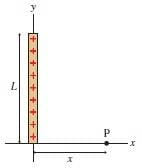# Find electric field a distance P from a charged rod

• isukatphysics69
The correct solution is:In summary, the electric field at point P is 15,000 N/C in the x-direction and 43,301 N/C in the y-direction.

## Homework Statement

The figure below shows a thin, vertical rod of length L with total charge Q. The indicated point P is a horizontal distance x from the one end of the rod. What is the electric field at point P. Express your answer in component notation in the two blanks below.

L = 5.0 cm, Q = 3.0 nC, and x = 3.0 cm.kq/r^2

## The Attempt at a Solution

theta = arctan(5/3) = 59 deg
total charge = [(8.99x10^9)(3x10^-9)]/((0.03)^2) = 29966
x component = 29966cos59=15417

1.8/2 points

#### Attachments

Last edited:
Nevermind I just forgot to square the distance lol

Why am I only getting 1.8/2 points for 15417N/C? is something incorrect?

this is so frustrating what is the sig figs ??

LOL IT WAS ROUNDED DOWN TO 15000

isukatphysics69 said:
LOL IT WAS ROUNDED DOWN TO 15000
So you're good now?•isukatphysics69
berkeman said:
So you're good now?yes please see other thread I am stuck again on y component

isukatphysics69 said:

## Homework Statement

The figure below shows a thin, vertical rod of length L with total charge Q. The indicated point P is a horizontal distance x from the one end of the rod. What is the electric field at point P. Express your answer in component notation in the two blanks below.

L = 5.0 cm, Q = 3.0 nC, and x = 3.0 cm.

View attachment 230884

kq/r^2

## The Attempt at a Solution

theta = arctan(5/3) = 59 deg
total charge = [(8.99x10^9)(3x10^-9)]/((0.03)^2) = 29966
x component = 29966cos59=15417

1.8/2 points
This working of this problem is incorrect.